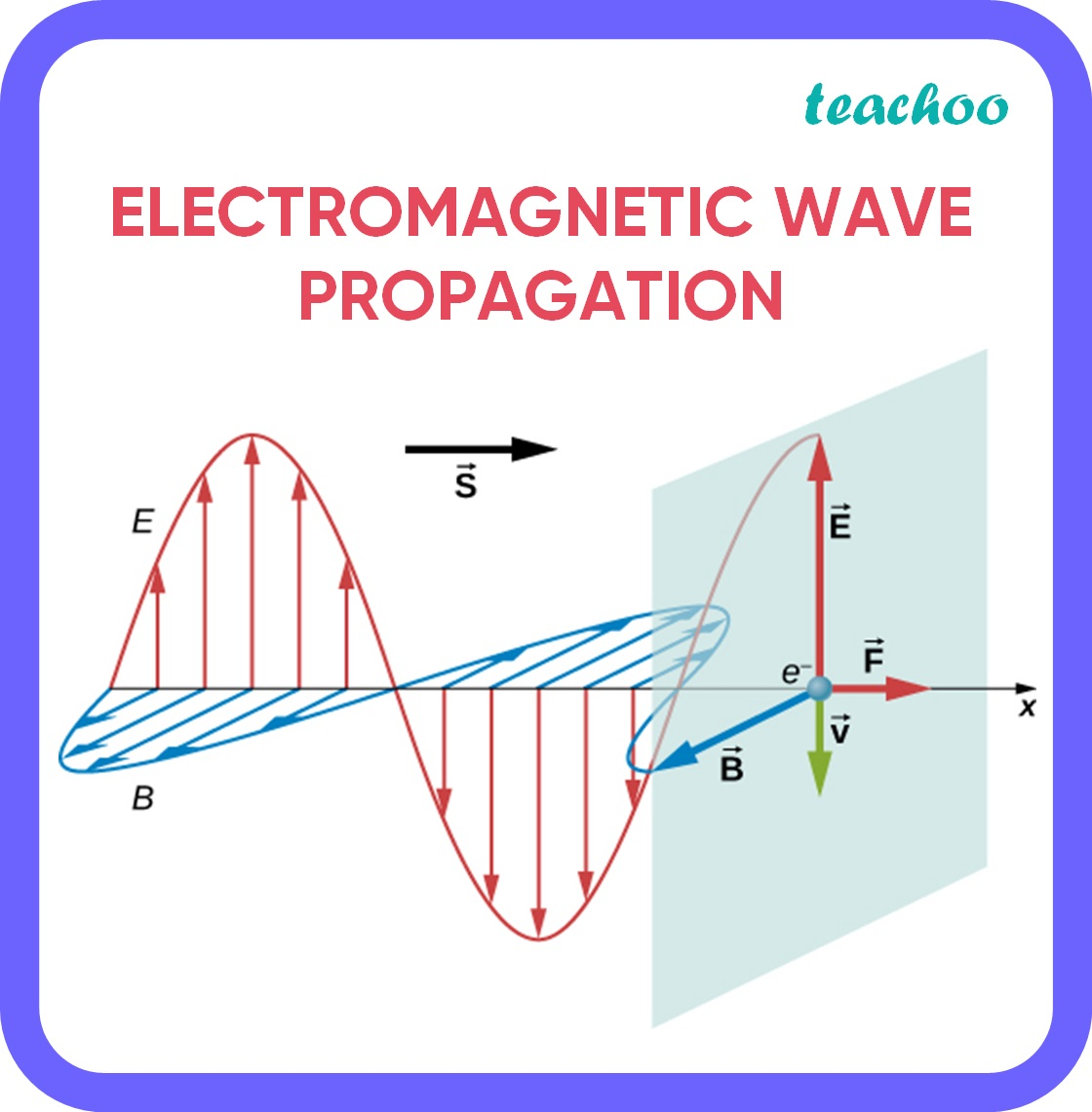CBSE Class 12 Sample Paper for 2022 Boards [Term 2] - Physics

Physics Class 12
Solutions to CBSE Sample Paper - Physics Class 12

## (c) An e.m. wave exerts pressure on the surface on which it is incident. Justify.

An EM Wave is composed of photons which have both energy (hv) and momentum (ƛ/h).

• We know that Pressure = Force per unit area.
• Force = Change in momentum per unit time.
• Therefore, Pressure = Δp/A Δt
• That is, pressure is exerted on surfaces due to a change in momentum .
• When an EM wave strikes a surface, its momentum is either absorbed or reflected , leading to a sudden change in momentum

This change in the momentum of EM Waves exerts pressure on surfaces .Learn in your speed, with individual attention - Teachoo Maths 1-on-1 Class